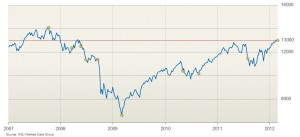# Mann Kendall Trend Test: Definition, Running the Test

Share on

Non Parametric Tests > Mann Kendall Trend Test

## What is the Mann Kendall Trend Test?The Mann Kendall Trend Test looks for trends in data. Dow Jones Timeplot from the Wall Street Journal.

The Mann Kendall Trend Test (sometimes called the M-K test) is used to analyze data collected over time for consistently increasing or decreasing trends (monotonic) in Y values. It is a non-parametric test, which means it works for all distributions (i.e. your data doesn’t have to meet the assumption of normality), but your data should have no serial correlation. If your data does follow a normal distribution, you can run simple linear regression instead.

The test can be used to find trends for as few as four samples. However, with only a few data points, the test has a high probability of not finding a trend when one would be present if more points were provided. The more data points you have the more likely the test is going to find a true trend (as opposed to one found by chance). The minimum number of recommended measurements is therefore at least 8 to 10.

## How the Test Works

• The null hypothesis for this test is that there is no monotonic trend in the series.
• The alternate hypothesis is that a trend exists. This trend can be positive, negative, or non-null.

Before running the test, you should ensure that:

1. Your data isn’t collected seasonally (e.g. only during the summer and winter months), because the test won’t work if alternating upward and downward trends exist in the data. Another test—the Seasonal Kendall Test—is generally used for seasonally collected data.
2. Your data does not have any covariates—other factors that could influence your data other than the ones you’re plotting. See Covariates for more information.
3. You have only one data point per time period. If you have multiple points, use the median value.

The Mann-Kendall Trend Test analyzes difference in signs between earlier and later data points. The idea is that if a trend is present, the sign values will tend to increase constantly, or decrease constantly. Every value is compared to every value preceding it in the time series, which gives a total of n(n – 1) / 2 pairs of data, where “n” is the number of observations in the set. For example, if you have 20 observations, the number of pairwise comparisons is:
20(20 – 1) / 2 = 20(19)/2 = 380/2 = 190.

As you can probably tell, even a relatively small data set can result in a huge number of comparisons. Although it’s possible to run the test by hand (you can find the steps in this Mann Kendall Analysis PDF), most people choose to use software. You have many options, including:

• R: Install the Kendall package developed by A.I. McLeod with the following command.
install.packages(“Kendall”)
Full instructions can be found in this Word document: Mann-Kendall Trend Test in R

Reference:
Prashanth Khambhammettu: ”Mann-Kendall Analysis for the Fort Ord Site”,
HydroGeoLogic, Inc.-OU-1 2004 Annual Groundwater Monitoring Report-Former Fort
Ord, California, 2005

CITE THIS AS:
Stephanie Glen. "Mann Kendall Trend Test: Definition, Running the Test" From StatisticsHowTo.com: Elementary Statistics for the rest of us! https://www.statisticshowto.com/mann-kendall-trend-test/
---------------------------------------------------------------------------Need help with a homework or test question? With Chegg Study, you can get step-by-step solutions to your questions from an expert in the field. Your first 30 minutes with a Chegg tutor is free!